# Representation theory

Representation theory is a branch of mathematics that studies abstract algebraic structures by representing their elements as linear transformations of vector spaces, and studies modules over these abstract algebraic structures. In essence, a representation makes an abstract algebraic object more concrete by describing its elements by matrices and their algebraic operations (for example, matrix addition, matrix multiplication). The theory of matrices and linear operators is well-understood, so representations of more abstract objects in terms of familiar linear algebra objects helps glean properties and sometimes simplify calculations on more abstract theories. The algebraic objects amenable to such a description include groups, associative algebras and Lie algebras. The most prominent of these (and historically the first) is the representation theory of groups, in which elements of a group are represented by invertible matrices in such a way that the group operation is matrix multiplication. Representation theory is a useful method because it reduces problems in abstract algebra to problems in linear algebra, a subject that is well understood. Furthermore, the vector space on which a group (for example) is represented can be infinite-dimensional, and by allowing it to be, for instance, a Hilbert space, methods of analysis can be applied to the theory of groups. Representation theory is also important in physics because, for example, it describes how the symmetry group of a physical system affects the solutions of equations describing that system. Representation theory is pervasive across fields of mathematics for two reasons. First, the applications of representation theory are diverse: in addition to its impact on algebra, representation theory: * illuminates and generalizes Fourier analysis via harmonic analysis, * is connected to geometry via invariant theory and the Erlangen program, * has an impact in number theory via automorphic forms and the Langlands program. Second, there are diverse approaches to representation theory. The same objects can be studied using methods from algebraic geometry, module theory, analytic number theory, differential geometry, operator theory, algebraic combinatorics and topology. The success of representation theory has led to numerous generalizations. One of the most general is in category theory. The algebraic objects to which representation theory applies can be viewed as particular kinds of categories, and the representations as functors from the object category to the category of vector spaces. This description points to two obvious generalizations: first, the algebraic objects can be replaced by more general categories; second, the target category of vector spaces can be replaced by other well-understood categories. (Wikipedia).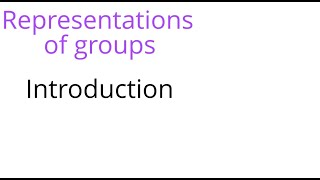Representation theory: Introduction

This lecture is an introduction to representation theory of finite groups. We define linear and permutation representations, and give some examples for the icosahedral group. We then discuss the problem of writing a representation as a sum of smaller ones, which leads to the concept of irr

From playlist Representation theory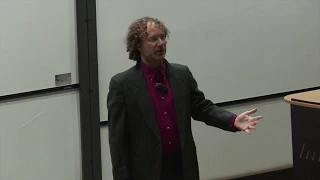Representation Theory as Gauge Theory - David Ben-Zvi 

Slides for this talk: https://drive.google.com/file/d/1FHl_tIOjp26vuULi0gkSgoIN7PMnKLXK/view?usp=sharing Notes for this talk: https://drive.google.com/file/d/1BpP2Sz_zHWa_SQLM6DC6T8b4v_VKZs1A/view?usp=sharing David Ben-Zvi (University of Texas, Austin) Title: Representation Theory as G

From playlist Number Theory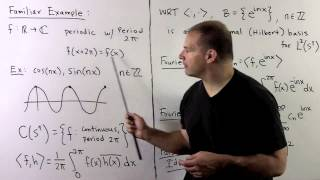RT6. Representations on Function Spaces

Representation Theory: We note how to transfer a group action of a group G on a set X to a group action on F(X), the functions on X. Because F(X) is a vector space, we obtain a representation of the group, and we can apply previous techniques. In particular, the group acts on itself in

From playlist Representation Theory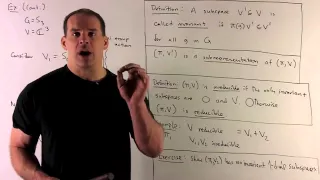RT1: Representation Theory Basics

Representation Theory: We present basic concepts about the representation theory of finite groups. Representations are defined, as are notions of invariant subspace, irreducibility and full reducibility. For a general finite group G, we suggest an analogue to the finite abelian case, whe

From playlist *** The Good Stuff ***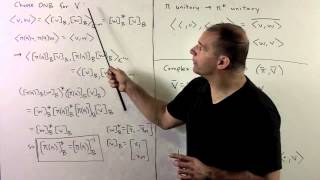RT4.1. Constructions from Linear Algebra (Expanded)

Representation Theory: We apply techniques from linear algebra to construct new representations from old ones. Constructions include direct sums, dual spaces, tensor products, and Hom spaces. Course materials, including problem sets and solutions, available at http://mathdoctorbob.org/U

From playlist Representation Theory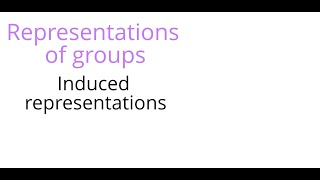Representation theory: Induced representations

We define induced representations of finite groups in two ways as either left or right adjoints of the restriction functor. We calculate the character of an induced representation, and give an example of an induced representation of S3.

From playlist Representation theory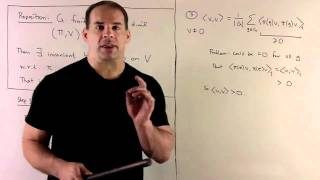RT2: Unitary Representations

Representation Theory: We explain unitarity and invariant inner products for representations of finite groups. Full reducibility of such representations is derived. Course materials, including problem sets and solutions, available at http://mathdoctorbob.org/UR-RepTheory.html

From playlist Representation Theory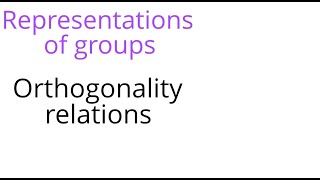Representation theory: Orthogonality relations

This lecture is about the orthogonality relations of the character table of complex representations of a finite group. We show that these representations are unitary and deduce that they are all sums of irreducible representations. We then prove Schur's lemma describing the dimension of t

From playlist Representation theory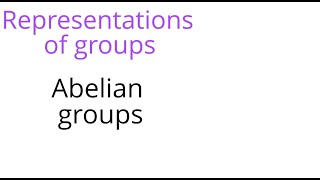Representation theory: Abelian groups

This lecture discusses the complex representations of finite abelian groups. We show that any group is iomorphic to its dual (the group of 1-dimensional representations, and isomorphic to its double dual in a canonical way (Pontryagin duality). We check the orthogonality relations for the

From playlist Representation theory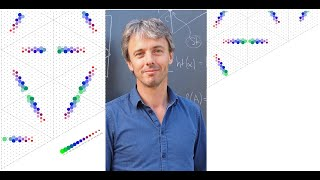Geordie Williamson 6 August 2020

Topic: Modular Representation Theory and Geometry Abstract: This will be a broad survey talk on interactions between geometry and representation theory, with a focus on representations in positive characteristic (“modular representation theory”). I will outline several basic questions (e.

From playlist Geordie Williamson external seminars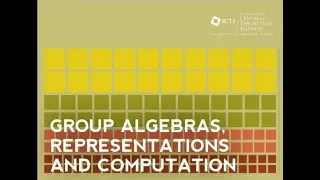Representations of finite groups of Lie type (Lecture 1) by Dipendra Prasad

PROGRAM : GROUP ALGEBRAS, REPRESENTATIONS AND COMPUTATION ORGANIZERS: Gurmeet Kaur Bakshi, Manoj Kumar and Pooja Singla DATE: 14 October 2019 to 23 October 2019 VENUE: Ramanujan Lecture Hall, ICTS Bangalore Determining explicit algebraic structures of semisimple group algebras is a fun

From playlist Group Algebras, Representations And Computation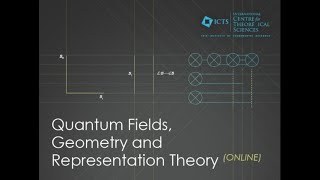A Mathematical Introduction to 3d N = 4 Gauge Theories (Lecture 1) by Mathew Bullimore

PROGRAM : QUANTUM FIELDS, GEOMETRY AND REPRESENTATION THEORY 2021 (ONLINE) ORGANIZERS : Aswin Balasubramanian (Rutgers University, USA), Indranil Biswas (TIFR, india), Jacques Distler (The University of Texas at Austin, USA), Chris Elliott (University of Massachusetts, USA) and Pranav Pan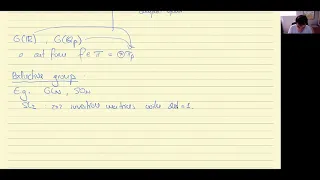Representations of p-adic reductive groups by Tasho Kaletha

PROGRAM ZARISKI-DENSE SUBGROUPS AND NUMBER-THEORETIC TECHNIQUES IN LIE GROUPS AND GEOMETRY (ONLINE) ORGANIZERS: Gopal Prasad, Andrei Rapinchuk, B. Sury and Aleksy Tralle DATE: 30 July 2020 VENUE: Online Unfortunately, the program was cancelled due to the COVID-19 situation but it will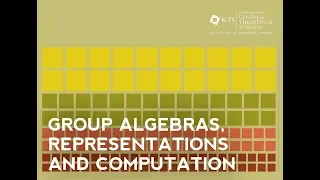On a Hecke algebra isomorphism of Kazhdan by Radhika Ganapathy

PROGRAM GROUP ALGEBRAS, REPRESENTATIONS AND COMPUTATION ORGANIZERS: Gurmeet Kaur Bakshi, Manoj Kumar and Pooja Singla DATE: 14 October 2019 to 23 October 2019 VENUE: Ramanujan Lecture Hall, ICTS Bangalore Determining explicit algebraic structures of semisimple group algebras is a fund

From playlist Group Algebras, Representations And Computation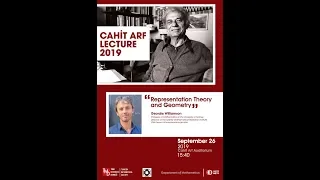Arf Lecture 2019 by Geordie Williamson

Arf Lecture 2019 was given by Geordie Williamson from The University of Sydney on September 26 at 15.40 in Cahit Arf Auditorium. Title: Representation theory and geometry Abstract: One of the most fundamental questions in representation theory asks for a description of the simple represe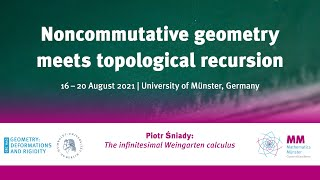Piotr Sniady: Representation theory from the random matrix perspective

Talk at the conference "Noncommutative geometry meets topological recursion", August 2021, University of Münster. Abstract: In many cases a representation of a group can be viewed as a "random matrix with non-commutative entries". This viewpoint gives a heuristic explanation for many links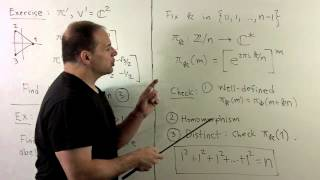RT3. Equivalence and Examples (Expanded)

Representation Theory: We define equivalence of representations and give examples of irreducible representations for groups of low order. Then we use the commutator subgroup to characterize all one dimensional representations of G (characters) in terms of the abelianization of G. Course

From playlist Representation Theory